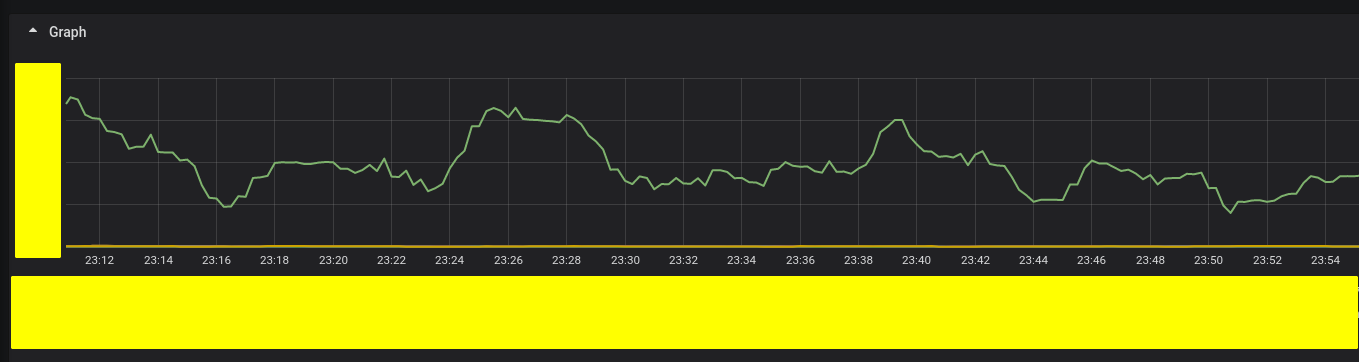# PromQL Guide

2020.10.02

# 文档翻译系列 XD

PromQL 全称 Prometheus Query Language, 是在 Prometheus 上使用的 DSL .

## Example

``````rate(web_request_count{status="200"}[5m]) >0
``````

``````rate(
web_request_count{status="200"}[5m]
) > 0
``````

`rate` 是 一个 PromQL 提供的函数, 专门用来计算 增长率, 常用于计算 QPS.

``````# 为了让命令确实可以运行, 这里替换成了 prometheus 自带的 go gc 监控, 不过意思是一样的
# URL 中没有 指定时间范围, 默认返回当前时间的瞬时数据
\$ curl 'http://localhost:9090/api/v1/query?query=rate(go_gc_duration_seconds_sum[5m])>0'
#                                                ^^^^^^^^^^^^^^^^^^^^^^^^^^^^^^^^^^^^^^ 这一块是实际运行的表达式
``````

``````{
"status": "success",
"data": {
"resultType": "vector",
"result": [
{
"metric": {
"instance": "127.0.0.1:9090",
"job": "prometheus",
"source": "k8s"
},
"value": [
1601183521.796,
"0.000004714821924357098"
]
}
]
}
}
``````## 格式

### PromQL 格式

• metrics 指标名
• labels
• 运算符和关键字
• 常量
• 时间范围选择器
``````sum(rate(go_gc_duration_seconds_sum{instance=~"\$instance",job=~"\$job"}[2m])) by (instance) > 0

avg(avg_over_time(up{instance=~"\$instance",job=~"\$job"}[\$interval]) * 100)

topk(20,sum(delta(api_time_use_count{code="200"}[\$__interval])) by (code)) >0
``````

### Result 格式

• 瞬时向量
• 某一个瞬时查询到的全部数据,例如 上面 Demo 演示的 例子
• 范围向量
• 一段时间内的 查询到的全部数据, 例如上面展示在 Grafana 中的例子
• 常量
• 一个运算结果, 例如上面 使用 avg 计算 启动率
• 字符串(暂未投入使用)

## 运算符和关键字

### 运算符

PromQL 对 基本的 运算符号都支持 , 例如

• `加减乘除+-*/`
• `求余%`
• `乘方^`
• `==` `!=` `<` `>` `>=` `<=` `正则匹配 =~`

### 关键字

PromQL 中的关键字很少,只有如下六个, 通常都用在处理 `Label``Join 操作` 上, 而这六个关键字又可以 又可以分为两类

• 在运算时忽略 label
• `without``by`
• 连接 (Join) 操作
• `ignoring``on`
• `group_right``group_left`

#### 在运算中 忽略 或者 仅关注 某些 Label

• `without``by` 只能用于单个 Metrics 的 函数运算中, 忽略 或者 仅关注 某些 label, 效果类似于 SQL 的 `group` 操作
``````// example about by , without 也是类似

// A{method="post", code="200",service="FooServer"}  24
// A{method="get", code="200",service="FooServer"}  30
// A{method="put", code="200",service="BarServer"}  321
// A{method="delete", code="200",service="BarServer"}  123

// 计算所有的请求量

// Before
sum(A{code="200"})
//
// SQL like this
// SELECT sum(value) FROM A,B
//
// result
// sum(A{code="200"}) 498

// After
sum(A{code="200"}) by (service)
// {service="BarServer"} 444
//
// SQL like this:
// SELECT sum(value), service FROM A,B GROUP BY service
//
// result
// {service="FooServer"} 54

// 当然, 读者也可以自行尝试 by (method) 来得到类似的结果
``````

#### Join

• `ignoring``on` 则用在 `两个 Metrics 做基础运算` 的场景中 忽略 或者 仅仅关注 某些 label, 你也可以理解成
``````// A{method="get", code="500"}  24
// A{method="get", code="404"}  30
// A{method="put", code="501"}  3
// A{method="post", code="500"} 6
// A{method="post", code="404"} 21

// B{method="get"}  600
// B{method="del"}  34
// B{method="post"} 120

A{code="500"} /  on(method) B
//
// SQL like this
// SELECT A.value * B.value, A.method
// FROM A INNER JOIN B ON (A.code = 500 AND A.method == B.method)
//
// result
// {method="get"}  0.04            //  24 / 600
// {method="post"} 0.05            //   6 / 120
// 而 method 等于 del 和 put 的由于找不到匹配项, 所以不会出现在结果中.

// <向量表达式> <二元运算符> ignoring(<Labels>) <向量表达式>
// <向量表达式> <二元运算符> on(<Labels>) <向量表达式>
``````
• 还可以将 `ignoring` /`on``group_left` 或者 `group_right` 相组合, 达成更细节的 join 效果, 当 `ignoring` /`on` 和 group 组合的时候, 他们的动作会稍微有所改变
``````// <向量表达式> <二元运算符> ignoring(<labels>) group_left(<labels>) <向量表达式>
// <向量表达式> <二元运算符> ignoring(<labels>) group_right(<labels>) <向量表达式>
// <向量表达式> <二元运算符> on(<labels>) group_left(<labels>) <向量表达式>
// <向量表达式> <二元运算符> on(<labels>) group_right(<labels>) <向量表达式>

// A{method="get", code="500"}  24
// A{method="get", code="404"}  30
// A{method="put", code="501"}  3
// A{method="post", code="500"} 6
// A{method="post", code="404"} 21

// B{method="get"}  600
// B{method="del"}  34
// B{method="post"} 120

A / on(method) group_left B
//
// SQL like this
// SELECT A.value * B.value, A.*
// FROM A INNER JOIN B ON (A.method == B.method)
//
// result
// {method="get", code="500"}  0.04            //  24 / 600
// {method="get", code="404"}  0.05            //  30 / 600
// {method="post", code="500"} 0.05            //   6 / 120
// {method="post", code="404"} 0.175           //  21 / 120
``````
• 除此之外, 还可以使用 `and` `or` `unless` 这些关键字实现两个 Metrics 之间的 `交集` `并集` `差集` 的运算, 同样 `and`/`or`/`unless` 也可以用 ignoring 和 on 修饰.

## 函数

PromQL 提供的函数很多 , 详细可以参考 官方文档来查看, 下面仅仅列举一些常用的函数和使用示例:

• rate / irate
``````// 求增长率

// rate 和 irate 的区别,
// irate 适合快速变化的计数器（counter），而 rate 适合缓慢变化的计数器（counter）。
``````
• delta / idelta / increase

计算 设定时间范围内的 第一个值减去最后一个值的 差, 例如 下面这个 表达式

``````// 每一分钟有多少请求
delta(web_request_total[1m]) // count 类型
``````
• `sum` / `avg` / `max` / `min`

• `sum_over_time` / `avg_over_time` / `max_over_time` / `min_over_time`

一段时间内的 sum 以及其他

• topk

• count

metrics 数量

• log2 / log10

常用于突出 底部的曲线变化,

• label_join

label 的修改

• label_replace

label 替换

• predict_linear

• ……

## Common collocation 常见搭配

### QPS

``````// 根据 count 类型的指标
rate(http_requests_total[5m])
``````

### 实际请求量

``````// 根据 count 类型指标
delta(http_requests_total[5m])
``````

### Histogram 区间内的请求量

``````// 根据 histogram 类型指标
sum(increase(http_requests_time_histogram_bucket{le="200"}[5m]))
-
sum(increase(http_requests_time_histogram_bucket{le="100"}[5m]))
``````

### 对标签使用正则匹配

``````http_requests_total{status_code=~"2.*"}
``````